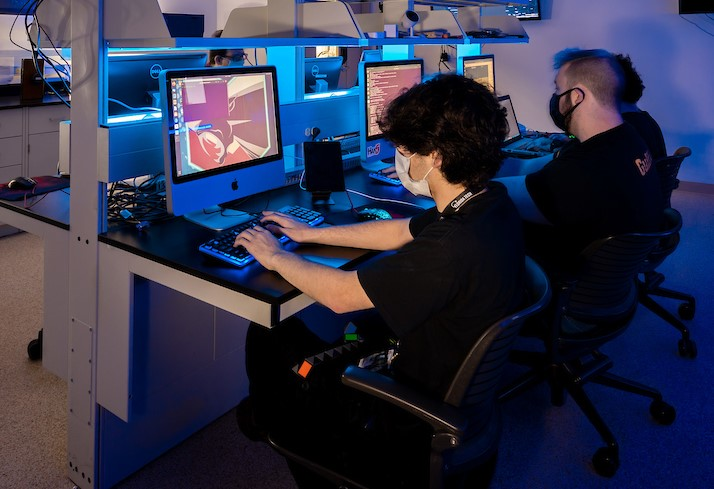## Electrical Engineering and Computer Science Faculty Publications

### Minimizing N-Points Interpolation Curvature, Heuristics for Solutions Using Arcs and Lines

Report

#### Abstract

Knowing a set of points on a curve, the interpolation problem is to hypothesize the location of the intermediary ones. A large set of interpolation techniques are known. We address the problem of generating a path with minimal maximum curvature, passing through N ordered points and joining the two end-points at predefined directions. This is related to R-geodesics, which have been used to generate paths with minimum average curvature between two given points that have to be joined at predefined directions and curvature. For example, when interpolating GPS points to reconstruct a vehicle’s trajectory, we may know that the centripetal acceleration is upper bounded due to physical constraints, hence adding constraints on the trajectory curvature. Among two interpolations with the same maximum curvature, we prefer the one with shorter trajectory. We compare experimentally several interpolations techniques, and propose heuristics to generate paths based on concatenated arc and line segments (also known as R-geodesics) inferred based on tuples of three consecutive points. Benchmarks with over 1000 simulated and real scenarios show that this algorithm is 73% percent better then the next candidate method we propose and which is based on bi-arcs with hillclimbing. A remaining open question is whether a global optima can be achieved and proven.

2-11-2013

COinS